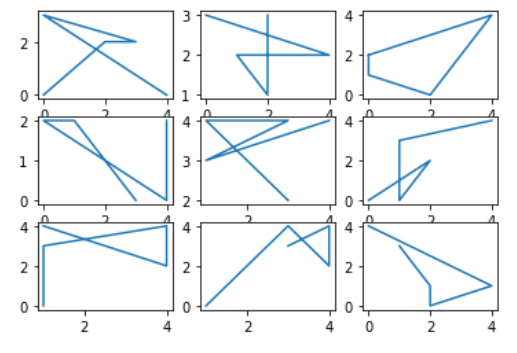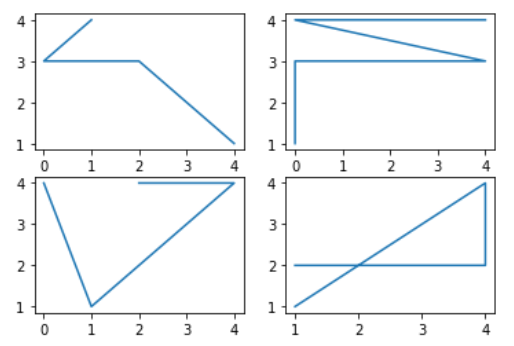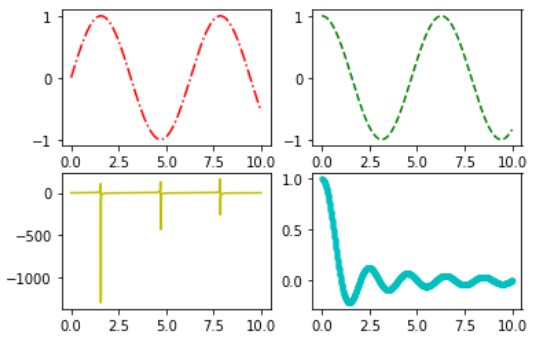Related Articles

# How to Create Subplots in Matplotlib with Python?

• Last Updated : 12 Nov, 2020

Prerequisite: Matplotlib

In this article, we will learn how to add markers to a Graph Plot using Matplotlib with Python. For that one must be familiar with the following concepts:

• Matplotlib : Matplotlib is a tremendous visualization library in Python for 2D plots of arrays. Matplotlib may be a multi-platform data visualization library built on NumPy arrays and designed to figure with the broader SciPy stack. It was introduced by John Hunter within the year 2002.
• Subplots : The matplotlib.pyplot.subplots() method provides a way to plot multiple plots on a single figure. Given the number of rows and columns, it returns a tuple (fig, ax), giving a single figure fig with an array of axes ax.

### Approach

• Import packages
• Import or create some data
• Create subplot objects.
• Draw a plot with it.

Example 1:

## Python3

 `# importing packages``import` `matplotlib.pyplot as plt``import` `numpy as np`` ` `# making subplots objects``fig, ax ``=` `plt.subplots(``3``, ``3``)`` ` `# draw graph``for` `i ``in` `ax:``    ``for` `j ``in` `i:``        ``j.plot(np.random.randint(``0``, ``5``, ``5``), np.random.randint(``0``, ``5``, ``5``))`` ` `plt.show()`

Output :Example 2 :

## Python3

 `# importing packages``import` `matplotlib.pyplot as plt``import` `numpy as np`` ` `# making subplots objects``fig, ax ``=` `plt.subplots(``2``, ``2``)`` ` `# draw graph``ax[``0``][``0``].plot(np.random.randint(``0``, ``5``, ``5``), np.random.randint(``0``, ``5``, ``5``))``ax[``0``][``1``].plot(np.random.randint(``0``, ``5``, ``5``), np.random.randint(``0``, ``5``, ``5``))``ax[``1``][``0``].plot(np.random.randint(``0``, ``5``, ``5``), np.random.randint(``0``, ``5``, ``5``))``ax[``1``][``1``].plot(np.random.randint(``0``, ``5``, ``5``), np.random.randint(``0``, ``5``, ``5``))`` ` `plt.show()`

Output :Example 3 :

## Python3

 `# importing packages``import` `matplotlib.pyplot as plt``import` `numpy as np`` ` `# making subplots objects``fig, ax ``=` `plt.subplots(``2``, ``2``)`` ` `# create data``x ``=` `np.linspace(``0``, ``10``, ``1000``)`` ` `# draw graph``ax[``0``, ``0``].plot(x, np.sin(x), ``'r-.'``)``ax[``0``, ``1``].plot(x, np.cos(x), ``'g--'``)``ax[``1``, ``0``].plot(x, np.tan(x), ``'y-'``)``ax[``1``, ``1``].plot(x, np.sinc(x), ``'c.-'``)`` ` `plt.show()`

Output :Attention geek! Strengthen your foundations with the Python Programming Foundation Course and learn the basics.

To begin with, your interview preparations Enhance your Data Structures concepts with the Python DS Course. And to begin with your Machine Learning Journey, join the Machine Learning – Basic Level Course

My Personal Notes arrow_drop_up